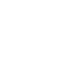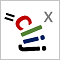# CLIJ2GPU accelerated image processing for everyone

CLIJ2 home

## drawTwoValueLineDraws a line between two points with a given thickness.

Pixels close to point 1 are set to value1. Pixels closer to point 2 are set to value2 All pixels other than on the line are untouched. Consider using clij.set(buffer, 0); in advance.

Availability: Available in Fiji by activating the update sites clij and clij2. This function is part of clijx_-0.32.0.1.jar.

### Usage in ImageJ macro

``````Ext.CLIJx_drawTwoValueLine(Image destination, Number x1, Number y1, Number z1, Number x2, Number y2, Number z2, Number thickness, Number value1, Number value2);
``````

### Usage in object oriented programming languages

Java
```// init CLIJ and GPU
import net.haesleinhuepf.clijx.CLIJx;
import net.haesleinhuepf.clij.clearcl.ClearCLBuffer;
CLIJx clijx = CLIJx.getInstance();

// get input parameters
destination = clijx.create();
float x1 = 1.0;
float y1 = 2.0;
float z1 = 3.0;
float x2 = 4.0;
float y2 = 5.0;
float z2 = 6.0;
float thickness = 7.0;
float value1 = 8.0;
float destination0 = 9.0;
```
```// Execute operation on GPU
clijx.drawTwoValueLine(destination, x1, y1, z1, x2, y2, z2, thickness, value1, destination0);
```
```// show result
destinationImagePlus = clijx.pull(destination);
destinationImagePlus.show();

// cleanup memory on GPU
clijx.release(destination);
```
Matlab
```% init CLIJ and GPU
clijx = init_clatlabx();

% get input parameters
destination = clijx.create();
x1 = 1.0;
y1 = 2.0;
z1 = 3.0;
x2 = 4.0;
y2 = 5.0;
z2 = 6.0;
thickness = 7.0;
value1 = 8.0;
destination0 = 9.0;
```
```% Execute operation on GPU
clijx.drawTwoValueLine(destination, x1, y1, z1, x2, y2, z2, thickness, value1, destination0);
```
```% show result
destination = clijx.pullMat(destination)

% cleanup memory on GPU
clijx.release(destination);
```

Imprint